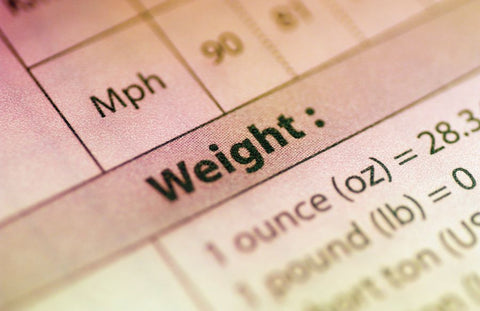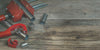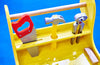# How to Convert Decimal Measurements to Fractions for DIY Projects

Converting decimal measurements to fractions is a crucial skill for DIY enthusiasts, handymen, and hobbyists. This article provides a comprehensive guide on how to convert decimal measurements to fractions for various DIY projects, from beginner to expert level.

We'll discuss the importance of understanding both Metric and SAE units in DIY projects, provide detailed examples, and offer clear guidelines to make the conversion process a breeze.

1. Understanding Decimal and Fractional Measurements
2. Why Convert Decimals to Fractions?
3. Metric and SAE Units in DIY
4. Methods for Converting Decimals to Fractions
1. Manual Conversion
2. Using Online Tools
3. Conversion Charts
5. Tips for Accurate Conversion
6. Examples of Decimal to Fraction Conversion in DIY Projects
7. Conclusion

## Understanding Decimal and Fractional Measurements

Before diving into the conversion process, it's essential to understand the difference between decimal and fractional measurements:

• Decimal measurements are based on the decimal number system, which uses a base of 10. They're expressed as decimals (e.g., 0.5 inches) and are commonly used in engineering, science, and computing.
• Fractional measurements are expressed as a ratio of two numbers, the numerator and the denominator (e.g., 1/2 inch). They're widely used in everyday life, especially in DIY projects, woodworking, and construction.

## Why Convert Decimals to Fractions?

Converting decimals to fractions is necessary for several reasons:

• Compatibility: Many DIY tools, such as tape measures and rulers, use fractional measurements. Converting decimals to fractions helps ensure that your measurements are compatible with these tools.
• Precision: Fractional measurements can provide greater precision than decimals, especially when dealing with small increments. This can be essential for achieving accurate results in your DIY projects.
• Ease of understanding: For many people, fractional measurements are more intuitive and easier to visualize than decimals. By converting decimals to fractions, you can better understand the dimensions of your project and communicate them to others.

## Metric and SAE Units in DIY

Both Metric and SAE (Imperial) units are commonly used in DIY projects. It's crucial to understand the differences between these systems and how they relate to decimal and fractional measurements:

• Metric system: The metric system is based on the meter, kilogram, and second, and uses a decimal system for all measurements. In this system, measurements are expressed in decimals (e.g., 12.7 millimeters).
• SAE system: The SAE (Society of Automotive Engineers) system, also known as the Imperial system, uses inches, feet, and pounds. In this system, measurements are typically expressed as fractions (e.g., 1/2 inch).

When converting decimal measurements to fractions, it's essential to determine which unit system you're working with. Keep in mind that some DIY projects may require you to convert between Metric and SAE units as well.

Related Article: Guide to Using a Laser Distance Measure

## Methods for Converting Decimals to Fractions

There are several methods for converting decimals to fractions, each with its advantages and disadvantages. In this section, we'll discuss three popular methods: manual conversion, using online tools, and conversion charts.

### Manual ConversionManual conversion involves using mathematical techniques to convert decimal measurements to fractions. Here's a step-by-step guide on how to do this, along with some examples in a DIY context:

1. Identify the decimal number: Locate the decimal number you want to convert to a fraction (e.g., 0.75 inches).
2. Multiply by a power of 10: Multiply the decimal number by a power of 10 to eliminate the decimal point (e.g., 0.75 x 100 = 75). The power of 10 you choose will become the denominator of your fraction.
3. Simplify the fraction: If necessary, simplify the resulting fraction by dividing both the numerator and the denominator by their greatest common divisor (e.g., 75/100 = 3/4).

Example 1: Cutting plywood for shelving

Suppose you're cutting plywood for shelves, and you need to make a cut at 0.625 inches. To convert this decimal measurement to a fraction:

1. Identify the decimal number: 0.625 inches.
2. Multiply by a power of 10: 0.625 x 1000 = 625.
3. Simplify the fraction: 625/1000 = 5/8.

So, you need to make a cut at 5/8 inch.

Example 2: Drilling holes for dowel joints

You have a dowel jig that requires you to drill holes at 0.875 inches apart. To convert this decimal measurement to a fraction:

1. Identify the decimal number: 0.875 inches.
2. Multiply by a power of 10: 0.875 x 1000 = 875.
3. Simplify the fraction: 875/1000 = 7/8.

So, you need to drill holes at 7/8 inch intervals.

Example 3: Measuring a gap for weatherstripping

You're installing weatherstripping around a window and need to measure the gap between the window frame and the sash. Your digital caliper shows a measurement of 0.1875 inches. To convert this decimal measurement to a fraction:

1. Identify the decimal number: 0.1875 inches.
2. Multiply by a power of 10: 0.1875 x 10000 = 1875.
3. Simplify the fraction: 1875/10000 = 3/16.

So, the gap measures 3/16 inch, and you can select the appropriate weatherstripping size accordingly.

### Using Online ToolsSeveral online tools can help you convert decimal measurements to fractions quickly and accurately. These tools typically require you to enter the decimal number and select the unit system (Metric or SAE) you're working with. They'll then provide you with the corresponding fractional measurement.

While online tools are convenient and easy to use, they may not always be available when you're working on a DIY project. It's a good idea to familiarize yourself with manual conversion techniques and have a backup method in place.

### Conversion ChartsConversion charts are another helpful tool for converting decimals to fractions. These charts list common decimal measurements and their corresponding fractional equivalents. To use a conversion chart, simply locate the decimal number you want to convert and find the corresponding fraction.

Keep in mind that conversion charts may not include every possible decimal measurement. In these cases, you'll need to use manual conversion techniques or an online tool.

## Tips for Accurate Conversion

When converting decimals to fractions, it's essential to ensure that your results are accurate and precise. Here are some tips to help you achieve this:

• Round decimals appropriately: When working with decimal measurements, ensure that you round them to the appropriate number of decimal places before converting them to fractions. This will help you maintain accuracy and precision in your DIY projects.
• Simplify fractions: Always simplify your fractions to their lowest terms. This will make them easier to understand and work with.
• Double-check your work: After converting a decimal measurement to a fraction, double-check your work to ensure that you haven't made any errors. This can help you avoid costly mistakes in your DIY projects.

## Examples of Decimal to Fraction Conversion in DIY Projects

Here are some examples of how decimal to fraction conversion might come into play in various DIY projects:

• Woodworking: When cutting pieces of wood for a project, you may need to convert decimal measurements (e.g., from a digital caliper) to fractions to accurately measure and mark your cuts using a tape measure or ruler.
• Plumbing: When working with pipe sizes, you may encounter decimal measurements (e.g., 0.5 inches) that need to be converted to fractions (e.g., 1/2 inch) to ensure compatibility with fittings and other components.
• Electrical work: When calculating wire sizes, you may need to convert decimal measurements (e.g., wire diameter) to fractions to determine the appropriate wire gauge for your project.

## Conclusion

Converting decimal measurements to fractions is an essential skill for DIY enthusiasts, handymen, and hobbyists.

By familiarizing yourself with the different methods of conversion, understanding the importance of Metric and SAE units, and following the tips provided in this article, you'll be well-equipped to tackle any DIY project that requires precise and accurate measurements.

Remember to always double-check your work and practice your conversion skills regularly to ensure success in your DIY endeavors.

### Related Topics##### Spring Clamps 101: What You Need to Know
Spring clamps, also known as pinch clamps or hand clamps, are indispensable tools for DIY enthusi...##### Everything You Need to Know About Pipe Clamps
Pipe clamps are an essential tool for DIY enthusiasts, handymen, and professionals alike. They pr...##### Find the Perfect Kids Workbench for Your Child's DIY Projects
Whether you are a parent, teacher, or homeowner looking to create a fun and educational environme...